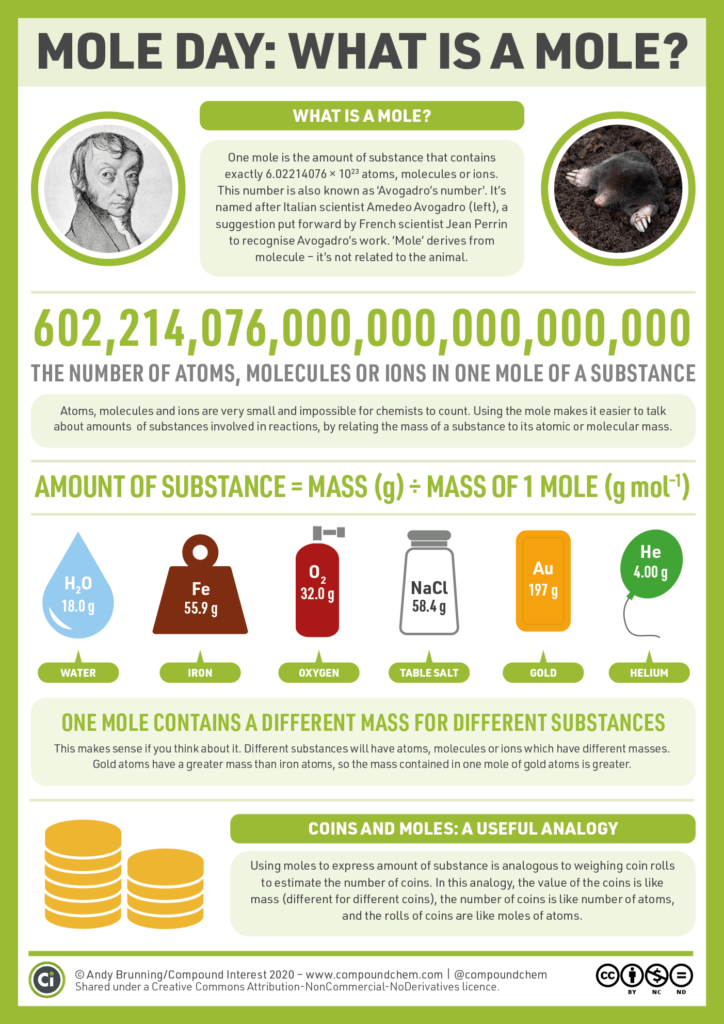## LEARNING OBJECTIVES

• Explain the energy content of a chemical reaction.
• Analyze the evidence of chemical change.
• Analyze the law of conservation of matter and how it applies to various types of chemical equations (synthesis, decomposition, single replacement, double replacement, and combustion).
• Analyze the stoichiometric relationships inherent in a chemical reaction.
• Analyze quantitatively the composition of a substance (empirical formula, molecular formula, percent composition, and hydrates).

## VIDEO

Crash Course on Stoichiometry

Introduction to the Mole

Calculating Molar Mass

Mole Calculations

Ratios & Proportions

Converting Grams to Moles

Basic Stoichiometry

Stoichiometric Calculations

Percent Composition

Empirical & Molecular Formulas

Hydrates

Calculating Percent Yield

## SIMULATIONS

PhET: Molarity

Gizmo: Stoichiometry

PhET: Limiting Reactants

Gizmo: Limiting Reactants

## WEBSITES

Reaction Stoichiometry

Mole Conversions Concept Builder

Stoichiometric Relationships Concept Builder